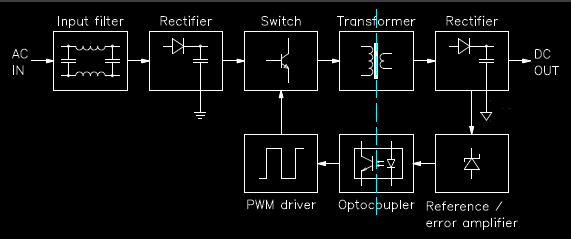# Switch Mode Power Supply Explained in Detail

A switch mode power supply, generally known as SMPS is an electronic device that is used to convert electrical power efficiently. This SMPS is more complex than conventional linear power supplies. The complexity increases but it give many advantages over the linear power supply.

## Why do we need a Linear Power Supply?

Well, this question must be clear before proceeding. All the electronic appliances (load) have different voltage and current ratings so we need to supply the electric power accordingly. This brings up the need for voltage regulators. They convert the mains supply voltage to the desired voltage of the particular electronic device.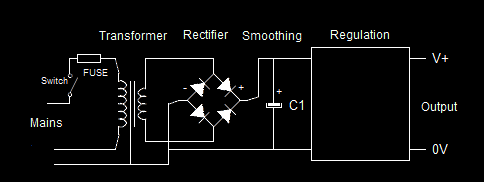Linear Power Supply

For this purpose, the Linear Voltage Regulator like the 78xx family is used most commonly as they are easy and simple to use. Let us understand with an example. Assume we have an electronic device that has a rated voltage rating of 5 volts. The ac mains supply has 230 volts which of course cannot be given directly to the device. Therefore, the mains supply is first fed to the linear voltage regulator (or maybe to an SMPS). Say an IC7805 is used as an LVR which converts the 230 volts to the 5 volts and then fed to the device.

Now, there are certain issues with these LVRs such as heating issues and efficiency. This makes the need for the switched-mode power supply. Let us have a look over the advantages and the disadvantages of the Linear Power Supply.

1. It is a simple and reliable system
2. Less ripple in the output voltage
3. Continuous power supply
4. This circuitry responds dynamically to load changes
5. Noise is low, as it is linear
6. Circuit resistance changes to regulate different the different output voltage

1. More heat dissipation
2. The transformers used are large and heavier
3. Efficiency is less, around 40-50%
4. Only a single voltage is obtained at the output

Therefore, all the devices which do not have the efficiency constraints and a simple regulation work there LPS fits well. Thus, to overcome the disadvantages of the linear power supply, the switched-mode power supply is introduced.

## Switch Mode Power Supply

The drawbacks like the lower efficiency, the need for filters (large capacitance) to cut the ripples, transformer, etc, are overcome by the use of switched-mode power supply.Switch Mode Power Supply

SMPS has become the most common and almost has replaced all the conventional ac-dc power supply everywhere. This is because SMPS is able to cut down on power consumption, size, and weight and less heat dissipation. Most electronic appliances that demand high efficiency incorporate the switched-mode power supply.

## Types of Switch Mode Power Supply

SMPS is classified on the basis of input and output voltages. The main classification is as follows:

• AC to DC
• DC to AC
• AC to AC
• DC to DC

Here in this, we are focusing on the first type of classification, i.e., AC to DC conversion.

## AC to DC SMPS Working

The basic AC to DC SMPS has the following components:

• Rectifier and filter (at input side)
• Inverter (with switching devices such as power MOSFET)
• A transformer
• Rectifier and filter (at the output)
• Feedback and control unit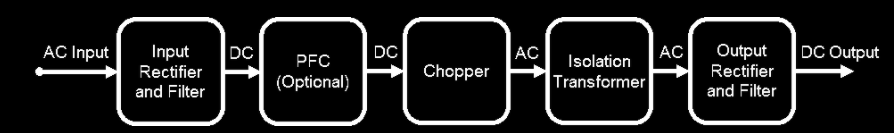The functioning of Switch Mode Power Supply

Let us now, now learn the functioning of all the above components one by one.

### Input Rectifier and Filter

A rectifier is a device that converts the ac signal to the dc signal. This operation is known as the rectification process. As we are considering ac to dc SMPS, so the input voltage would be an ac signal. This ac signal is then given to the rectifier circuit (maybe to the half-wave or the full-wave rectifier) where the signal is converted into the dc with ripples (and harmonics). The obtained waveform is also called the unregulated dc. This rippled dc is given to the filter, here a large value capacitor. This filter cuts down on the ripples or harmonics and makes the signal pure or near to pure dc.

An SMPS can be run from a dc supply as well as the dc signal will pass through the rectifier unaffected. But if the voltage supply has no voltage selector switch then it could create issues. For instance, if the power supply is designed for Vo volts ac then the dc voltage would be Vo*root 2. This is too harmful to the circuit as it produces heat which may permanently damage the components involved.

This issue could be resolved if we connect a voltage selector switch and keep the voltage as 2Vo. This gives the involved components (diodes) much freedom to handle the current as the voltage has been doubled now. Therefore, this is a safer way of operation.

### Inverter Block

So what is an Inverter?

This is a circuit that converts the dc signal to the ac signal. Let us understand it with a very practical example. You must have seen the inverters installed at your home. What does it do?

Yes, it gives us the supply when there is a power cut in the mains. It has battery storage where it stores the power and when needed it just converts the dc power (from the battery) into ac and then delivers. The same is the purpose here.

The input to this inverter is the direct dc from the input or the converted dc from the rectifier as explained above. Now the inverter is turned on and off at higher frequencies of 20kHz to 200kHz by the power transistors (power MOSFET).

### Transformer

After the inverter, the transformer is placed. Therefore, the high-frequency pulses from the inverter are given to the transformer primary. The rectified output is taken from the secondary of the transformer. This is then smoothed and produces the required DC voltage.

This transformer is unlike the 50, 60 Hz transformer and is light in weight and smaller in size.

### Feedback and Control Unit

The feedback circuit observes the output voltage and accordingly controls the dc waveform by changing the duty cycle. The feedback unit compares the output voltage with the reference and gives the instruction to the control unit.

## Non-Isolated Power Supplies

There are power supplies that do not contain transformers. They use inductors. Buck converters, boost converters, and buck-boost converters are examples of such types of power supplies. These converters are the simplest class of single input and a single output. These use one inductor and one active switch.

### Buck Mode Power Supply

Buck means to reduce. Therefore, the buck converter reduces the voltage from a higher value to the lower one. This converter does so without changing the polarity of the output voltage. Thus, we can call this converter the step-down regulator which can for example convert say +12 volts to +5 volts or so.Buck converter used in Switch Mode Power Supply

The buck converter is one of the simplest circuits which converts DC to DC voltage at different levels. It is used within a switch-mode power supply and uses power MOSFET or IGBT as the switching device.

#### The duty cycle of Buck Converter

The duty cycle is the ratio of the ON time divided by the total time.

Mathematically, D = Ton / (Ton + Toff)

Therefore, the output voltage of the buck converter can be given as:

Vout = (Ton / (Ton + Toff) ) * Vin

= D * Vin

So, the larger the duty cycle, the more the average DC output voltage. One more thing to notice here is that the output voltage will always be less than the input. This is because the on time will be less than the total time, as the total time consists of both on and off time.

Another advantage of this buck converter is that the inductor-capacitor is placed in such a manner that it filters the output voltage and makes it ripple-free. Ideally, the converter operates in continuous mode where the inductor current does not go to zero. In such an ideal case, the efficiency of the converter is 100% which is not at all practical.

### Boost Mode Power Supply

This is another type of switch-mode power supply that makes the given voltage to a higher value. The components of the boost converters are the same as that of the buck but are differently positioned.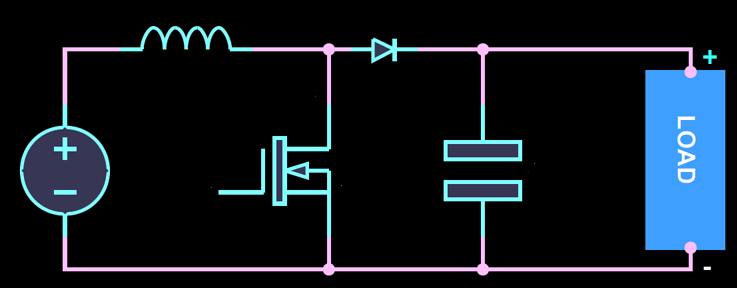Boost converter used in Switch Mode Power Supply

As the name suggests, the boost (to increase) converter increases the DC voltage from a lower to a higher value. Therefore, we can call it a step-up voltage regulator. So, any lower value, say 5 volts can be boosted up to 12 volts or so as per the requirement. Unlike the buck converter, it uses the parallel connection of the transistors to control the voltage of the output.

In the boost converter, when the transistor is on, the voltage flow is from supply to the inductor to the transistor and back to the supply. Thus, it does not go to the load when the transistor is switched ON as it behaves like the short circuit. By this, the inductor gets charged. Now, when the transistor is reverse biased then the input is connected to the load via the inductor and the diode, and the total voltage at the output becomes

Vout = Vin + VL

Therefore, we could see that the final output voltage is increased.

#### Duty Cycle of Boost Mode

The duty cycle is the same, i.e., the ON time by the total time. Therefore the output voltage can be written mathematically as:

Vout = (1 / (1 – D) ) Vin

So here again the output voltage can be controlled with the help of changing the duty cycle. The more the duty cycle the more the output voltage and vice-versa. This SMPS switching configuration is more commonly used in applications like photo flashes, battery chargers, strobe flashes, etc.

The interesting thing is that we can combine both the operations and the combo is called the buck-boost converter. Let us understand the operation in brief.

### Buck-Boost Power Supply

This combination of the buck and the boost converter produces an output that is negative and could be lesser or higher than the input voltage. This output voltage value depends upon the duty cycle and can be easily controlled by changing the duty cycle value.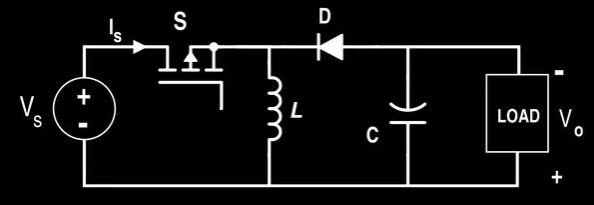Buck-Boost converter

When the switch is ON, the inductor gets charged and nothing goes to the load. Similarly, when the switch is OFF, then the inductor is discharged through the load and nothing comes from the supply voltage during this time. So the total output voltage at the output is the sum of the two and depending on the duty cycle, the output voltage is changed.

#### Output Voltage of Buck-Boost Converter

Mathematically, the output voltage of the buck-boost converter can be given as:

Vout = (D / (1 – D)) * Vin

From the equation, it is very clear that the output can be varied by varying the duty cycle of the signal. One thing to note here that is different from the two converters (individually) is that it gives the opposite polarity of the output voltage.

## Advantages of the Switched Mode Power Supply

• The efficiency is high, varying around 80-90%.

As switching action takes place continuously, therefore the device is either ON or OFF. This reduces the heat in the device and higher levels of efficiency can be achieved here.

• Compact: The size of SMPS is compact, simply because the size of the high-frequency transformers is smaller. Also, as the heat dissipation is less therefore, it can be made compact without thinking much of the hearing loss and efficiency.
• Flexible technology: We have seen that SMPS uses flexible technologies like a buck converter, boost converter, and the buck-boost converter. These are used to change the output voltage to either a higher or a lower value.
• Costs: This is one of the most attractive advantages of switch-mode power supply as it is much cheaper than the linear power supply. Again, as less heat so lesser the expenditure to reduce the heat and also the components get freedom and fewer constraints which makes them lesser in cost.

## Disadvantages of Switched Mode Power Supply

• Electromagnetic interference.
• As high-frequency switching is used, there are higher chances of noise.
• The circuit is complex when compared with the linear power supply.

## Applications of Switched Mode Power Supply

There are n numbers of applications of SMPS. Let us have a look at some common applications. These are used in:

• Laptops
• HVDC measurements
• Motor vehicles
• Motherboard of computers
• Space stations
• Security systems
• Central power distributions
• Consumer electronic devices and many more

Ajay Dheeraj

(Technical Content Developer)

•
•
•
•
•
•